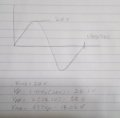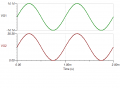# Circuit Analysis. Capacitor in AC and DC circuit.

#### VinceClortho

Joined Mar 30, 2016
20
Last edited by a moderator:

#### JoeJester

Joined Apr 26, 2005
4,390
You can begin by describing the AC signal ... Draw it out and lable the amplitudes

#### wayneh

Joined Sep 9, 2010
17,461
Your formula might be helpful if you define the terms better. What's Vmin? How do you get Vp? See Joe's comment.

#### VinceClortho

Joined Mar 30, 2016
20
Your formula might be helpful if you define the terms better. What's Vmin? How do you get Vp? See Joe's comment.
As I understand it Vmin is the minimum voltage of the sine wave. Vp is the peak value of the sine wave and is found by multiplying Vrms by 1.414.

#### Jony130

Joined Feb 17, 2009
5,475

#### JoeJester

Joined Apr 26, 2005
4,390
@VinceClortho

Review the terms RMS, peak, average, and peak to peak with respect to a sine wave signal.

Last edited:

#### VinceClortho

Joined Mar 30, 2016
20
Yes, but notice that -20V RMS ( -28.2V peak) will kill the electrolytic capacitor so you must "shift" this voltage up.
So does "shifting" it up mean introducing a DC voltage greater than peak? As I understand it that means the voltage will never reach negative.

#### VinceClortho

Joined Mar 30, 2016
20

#### Jony130

Joined Feb 17, 2009
5,475
So does "shifting" it up mean introducing a DC voltage greater than peak?
Yes, something like this.

#### VinceClortho

Joined Mar 30, 2016
20
@VinceClortho

Review the terms RMS, peak, average, and peak to peak with respect to a sine wave signal.
http://imgur.com/KYRiFa1 Here are some new values. I still don't quite understand where I went wrong, would you mind showing me?Last edited by a moderator:

#### MrAl

Joined Jun 17, 2014
10,871
Hi,

You want to make sure the capacitor voltage never goes negative.
The sine voltage goes negative without the DC voltage source, but with the DC source having the correct value the cap voltage will never go negative and thus the cap will be ok. Note the polarity of the cap. That means it cant go negative or the cap blows out.

Since you know the sine voltage, figure out what DC voltage it would take, when added to the sine voltage, to keep the cap voltage always positive.

#### VinceClortho

Joined Mar 30, 2016
20
Hi,

You want to make sure the capacitor voltage never goes negative.
The sine voltage goes negative without the DC voltage source, but with the DC source having the correct value the cap voltage will never go negative and thus the cap will be ok. Note the polarity of the cap. That means it cant go negative or the cap blows out.

Since you know the sine voltage, figure out what DC voltage it would take, when added to the sine voltage, to keep the cap voltage always positive.
Assuming I use the general rule of one standard unit larger I would think that 29.3 V would be correct. Vp=28.3; Vmin=Vdc-Vp= 29.3V-28.3V =1V. Is this correct?

#### JoeJester

Joined Apr 26, 2005
4,390
Here are some new values. I still don't quite understand where I went wrong, would you mind showing me?
I wanted you to realize the relationships illustrated in this graphicHere is a 1 kHz signal. what can you sat about them?Last edited:
•VinceClortho

#### VinceClortho

Joined Mar 30, 2016
20
I wanted you to realize the relationships illustrated in this graphicThank you very much for this and your help. Thanks everyone for the help.

#### WBahn

Joined Mar 31, 2012
29,469
@JoeJester Note that the Average Value for the green waveform shown is 0V. I think it is trying to get at the average value of the full-wave rectified version of the green waveform.

#### JoeJester

Joined Apr 26, 2005
4,390

#### MrAl

Joined Jun 17, 2014
10,871
Hi,

The mathematical average of a sine wave is zero, but the average voltage of a sine wave voltage source is taken to be 2/pi times the peak, which is about 0.637 times the peak, and this is correct even though it goes plus and minus and would normally average out to zero over any integer number of full cycles. Mathematically that is the average of abs(sin(wt)) which is the average of the absolute value of the sine wave which of course is the average of the ideal full wave rectified sine voltage.

#### WBahn

Joined Mar 31, 2012
29,469
Hi,

The mathematical average of a sine wave is zero, but the average voltage of a sine wave voltage source is taken to be 2/pi times the peak, which is about 0.637 times the peak, and this is correct even though it goes plus and minus and would normally average out to zero over any integer number of full cycles. Mathematically that is the average of abs(sin(wt)) which is the average of the absolute value of the sine wave which of course is the average of the ideal full wave rectified sine voltage.
Taken to be that by who (whom?)? I'm not being snide, I've just never seen (that I recall) the claim that the average voltage of a pure sine wave is anything other than zero (before the graphic that JoeJester posted, anyway). If I pass a signal through a low pass filter (of appropriately low cutoff frequency) I will see the average value of the waveform. If I pass a pure sine wave through such a filter I will see zero (or very nearly zero) at the output. I will NOT see 2/pi times the peak. A sine wave and a full-wave rectified sine wave are two fundamentally different waveforms.

#### JoeJester

Joined Apr 26, 2005
4,390
@MrAl , @WBahn

That graph illustrates the average position on that half cycle, not the whole wave. If we did the same for the negative half, it would end with a zero net sum.

I don't recall if my instructor from 1973 specified it was for just that half cycle. It was my mistake for not specifying the values were only for the half cycle specified on the graph.

Of course, if we connected an analog multi-meter to that signal, the signal is full wave rectified prior to the input of the meter circuit.

WBahn is correct because I failed to clarify that graph with text.